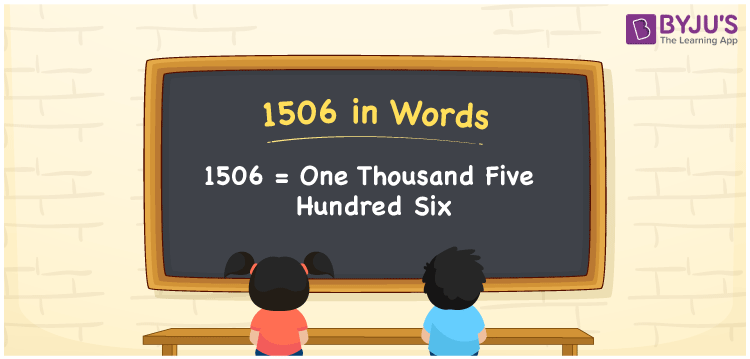# 1506 in words

1506 in words is written as One Thousand Five Hundred and Six. 1506 represents the count or value. The article on Counting Numbers can give you an idea about count or counting. The number 1506 is used in expressions that relate to money, distance, length, year and others. Let us consider an example for 1506. ”The tailor had to stitch One Thousand Five Hundred and Six suits for a wedding.”

 1506 in words One Thousand Five Hundred and Six One Thousand Five Hundred and Six in Numbers 1506

## 1506 in English Words## How to Write 1506 in Words?

We can convert 1506 to words using a place value chart. The number 1506 has 4 digits, so let’s make a chart that shows the place value up to 4 digits.

 Thousands Hundreds Tens Ones 1 5 0 6

Thus, we can write the expanded form as:

1 × Thousand + 5 × Hundred + 0 × Ten + 6 × One

= 1 × 1000 + 5 × 100 + 0 × 10 + 6 × 1

= 1506

= One Thousand Five Hundred and Six.

1506 is the natural number that is succeeded by 1505 and preceded by 1507.

1506 in words – One Thousand Five Hundred and Six.

Is 1506 an odd number? – No.

Is 1506 an even number? – Yes.

Is 1506 a perfect square number? – No.

Is 1506 a perfect cube number? – No.

Is 1506 a prime number? – No.

Is 1506 a composite number? – Yes.

## Solved Example

1. Write the number 1506 in expanded form

Solution: 1 × 1000 + 5 × 100 + 0 × 10 + 6 × 1

We can write 1506 = 1000 + 500 + 00 + 6

= 1 × 1000 + 5 × 100 + 0 × 10 + 6 × 1

## Frequently Asked Questions on 1506 in words

Q1

### How to write 1506 in words?

1506 in words is written as One Thousand Five Hundred and Six.
Q2

### Is 1506 a perfect square number?

No. 1506 is not a perfect square number.
Q3

### Is 1506 a prime number?

No. 1506 is not a prime number.